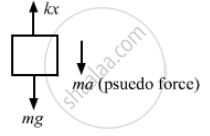# Suppose the Ceiling in the Previous Problem is that of an Elevator Which is Going up with an Acceleration of 2.0 M/S2. Find the Elongation. - Physics

Sum

Suppose the ceiling in the previous problem is that of an elevator which is going up with an acceleration of 2.0 m/s2. Find the elongation.

#### Solution

When the ceiling of the elevator is going up with an acceleration 'a', then a pseudo-force acts on the block in the downward direction.a = 2 m/s2

From the free-body diagram of the block,
kx = mg + ma
⇒ kx = 2g + 2a
= 2 × 9.8 + 2 × 2
= 19.6 + 4
$\Rightarrow x = \frac{23 . 6}{100} = 0 . 236 \approx 0 . 24 \text{ m }$
When 1 kg body is added,
total mass = (2 + 1) kg = 3 kg
Let elongation be x'.
∴ kx' = 3g + 3a = 3 × 9.8 + 6

$\Rightarrow x' = \frac{35 . 4}{100}$
$= 0 . 354 \approx 0 . 36 m$

So, further elongation = x' − x
= 0.36 − 0.24 = 0.12 m.
Concept: Newton’s Second Law of Motion
Is there an error in this question or solution?

#### APPEARS IN

HC Verma Class 11, Class 12 Concepts of Physics Vol. 1
Chapter 5 Newton's Laws of Motion
Q 18 | Page 80